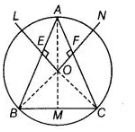# A, B and C are three points on a circle.`
Question:

A, B and C are three points on a circle. Prove that the perpendicular bisectors of AB, BC and CA are concurrent.
Thinking Process

1. Firstly, inscribe a ΔABC in a circle, then draw the perpendicular bisecters of any two sides of a triangle.
2. Secondly, prove that ΔOEA and ΔOEB are congruent by SAS rule and also ΔOMB and ΔOMC are congruent by RHS rule. Further, prove the required result.

Solution:

Given $A$ circle passing through three points $A, B$ and $C$.

Construction Draw perpendicular bisectors of $A B$ and $A C$ and they meet at a point $O$. Join $O A, O B$ and $O C$.

To prove Perpendicular bisector of $B C$, also passes through $O$ i.e., LO, ON and OM are concurrent.Proof In $\triangle O E A$ and $\triangle O E B$,

$A E=B E$

$[O L$ is the perpendicular bisector of $A B]$

$\angle A E O=\angle B E O$    [each 90°]

and    $O E=O E$    [common side]

$\therefore$ $\triangle O E A \equiv O E B$ [by SAS congruence rule]

$\therefore$ $O A=O B$

Similarly, $\Delta O F A \equiv \triangle O F C$ [by SAS congruence rule]

$\begin{array}{llll}\therefore & O A=O C & & \text { [by CPCT] }\end{array}$

$\therefore \quad O A=O B=O C=t \quad$ [say]

Now, we draw a perpendicular from $O$ to the $B C$ and join them. In $\triangle O M B$ and $\triangle O M C$,

$O B=O C$ [proved above]

$O M=O M$ [common side]

and $\quad \angle O M B=\angle O M C \quad$ [each $90^{\circ}$ ]

$\therefore \quad \Delta O M B \equiv \Delta O M C \quad$ [by RHS congruence rule]

$\Rightarrow$ $B M=M C$ [by CPCT]

Hence, $O M$ is the perpendicular bisector of $B C$.

Hence, $O L, O N$ and $O M$ are concurrent.

Hence proved.